### IMO Shortlist 2009 problem G2

Kvaliteta:
Avg: 3,0
Težina:
Avg: 6,0
Let$ABC$ be a triangle with circumcentre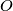$O$. The points$P$ and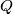$Q$ are interior points of the sides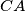$CA$ and$AB$ respectively. Let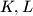$K,L$ and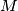$M$ be the midpoints of the segments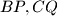$BP,CQ$ and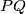$PQ$. respectively, and let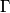$\Gamma$ be the circle passing through$K,L$ and$M$. Suppose that the line$PQ$ is tangent to the circle$\Gamma$. Prove that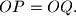$OP = OQ.$

Proposed by Sergei Berlov, Russia
Izvor: Međunarodna matematička olimpijada, shortlist 2009